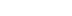/

Simple Physics-Based Motion with Springs

How do you move an object to a desired position by applying forces? With a Simple Physics-Based Position Controller

Originally posted here.

Simple Physics-Based Motion

How do you move from Point A to Point B with Physics? At times, you may want to compute the forces required to move an object from its current position to another position. This is not hard to do. It just requires some high school level knowledge of Physics, which we will review here.

If you want to move from point A to point B, it requires some forces to do so. Recall that F = ma. Where F is the force, m is the mass, and a is the acceleration. Also note that mass is a constant and that acceleration is the second time-derivative of position. Note that acceleration is the second time-derivative of position.

Unrealistic Motion with Velocity

Most people will implement a constant velocity to get from point A to point B, because this is simple. Note that velocity is the first time-derivative of position. You can either add a position-delta to the position parameter per simulation step,

while(bGameLoop == true) {
gameObject.position += positionDelta;
}

or you can simply set the velocity parameter to a constant value (if your system allows this).

gameObject.velocity = currentVelocity;

If you want to get to point B near-instantly, then you will use a very large velocity. If you want to animate motion from point A to point B, then you can use a smaller velocity. Either way, acceleration will be zero, in this case, since velocity is constant. This means that the motion will not be physically realistic, since there are no forces.

Realistic Motion with Acceleration

For a far more realistic animation of motion, you can use acceleration or forces. To simulate forces, we will use linear springs. A linear spring is expressed simply as

F = k * x

Where F is the force, k is the spring stiffness constant, and x is the object’s distance from its desired position. Note that x is a signed distance, and not an absolute magnitude.

To upgrade the accuracy of the linear spring from a first order equation to a second order equation, we can add damping into the equation. To do so we just need to add another term to the original equation.

F = k * x + d * v

Where d is the damping constant, and v is the object’s difference in velocity between its current velocity and its desired velocity.

The following is the simple function that you will need to compute the spring-based force from the object.

Vector3 getLinearSpringForce() {
Vector3 x_ij = newPos — currPos;
Vector3 v_ij = newVel — currVel;
Vector3 springForce = K * x_ij + D * v_ij;
return springForce;
}

That’s it!

Latest Jobs

Pocketwatch Games

Remote
01.05.22
Senior Gameplay Engineer

Disbelief

Cambridge, Massachusetts
01.07.22
Jr. Programmer

Gameloft Australia

Brisbane, Australia
01.06.21
Creative Director

Sucker Punch Productions

Bellevue, Washington
01.06.22
Senior Systems Designer
More Jobs